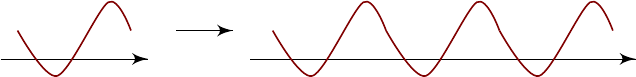2Fourier series

IB Methods2 Fourier series
The first type of functions we will consider is periodic functions.
Definition
(Periodic function)
.
A function
f
is periodic if there is some fixed
R such that f(x + R) = f(x) for all x.
However, it is often much more convenient to think of this as a function
f
:
S
1
C
from unit circle to
C
, and parametrize our function by an angle
θ
ranging from 0 to 2π.
Now why do we care about periodic functions? Apart from the fact that
genuine periodic functions exist, we can also use them to model functions on a
compact domain. For example, if we have a function defined on [0
,
1], we can
pretend it is a periodic function on
R
by making infinitely many copies of the
function to the intervals [1, 2], [2, 3] etc.
x x